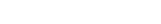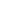Batch Test #4 Bull's Eye Plus Batch for JEE Mains & Advanced 2023Held on Dec 12, 2021
11:30 AM54 Q’s · 198 marks
180 minsLanguages
EnglishSyllabus

Mathematics
• Circles
• Statistics
• Properties of Triangle
• Set Theory
• Parabola
• Trigonometric Identities & Equations
• Theory of equations
• Complex Numbers
• Logarithm & its Properties
• Ellipse
• Basics & 2D System
• Binomial Theorem
• Series & Progressions
• Hyperbola
• Inequalities and Absolute Value
• Straight Lines

Chemistry
• Periodic Table and its Properties
• Alkenes & Alkynes Halides
• Acids and Bases
• Chemical Equilibrium
• General Topics & Mole Concept
• Aromatic Hydrocarbons
• Alkanes & Alkyl Halides
• Atomic Structure
• Basic Principle of Organic
• Stereochemistry & Isomerism
• Gases & Liquid States
• Hydrogen
• Chemical Bonding

Physics
• Motion in a Straight Line
• Motion in a Plane
• Work, Energy & Power
• Thermodynamics
• Uniform Circular Motion
• Vectors
• Laws of Motion & Friction
• Centre of Mass & Linear Momentum
• Gravitation
• Basic Mathematics
• Least Count Analysis
• Simple Harmonic Motion
• Kinetic Theory of Gases
• Rotational Motion
• Heating Effects of Current